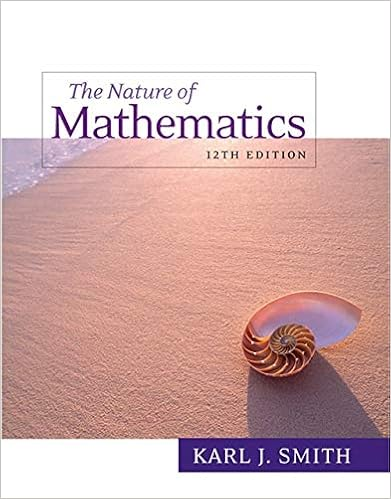# Week+2-Chapter+2+In+class+Activities+A+(1) - Math 040...

• 3

This preview shows page 1 - 3 out of 3 pages.

##### We have textbook solutions for you!
The document you are viewing contains questions related to this textbook.The document you are viewing contains questions related to this textbook.
Chapter 13 / Exercise 44
Nature of Mathematics
SmithExpert Verified
Math 040: Re-Entry Mathematics Franklin UniversityWeek 2 Chapter 2 In class ActivitiesName: ______________________________ 1.Fill in the blank with < or > to make a true statementa.- 8 - 9 b.- 226 1982.Find the oppositea.31 ___________b.-612 ___________3.Simplify the expressiona.– (-15) _________b.|79|________c.– (34) _________d.|47|_________4.Fill in the blank with <, >, or =a.– ( – 4) _____ |b.– 44 _______ – 54c.|55|______ – ( – 65) 5.Add the numbers a.23 + 12 __________b.78 + (-33) _________c.-105 + ( -23) ________d.-475 + (623) ________6.Simplifya.20 + (−12) + (−5) ______________b.−23 + (−4) + (−12) + (−5) ____________c.4 + (−12) + (−30) + 16 + 10 _____________7.A contestant on Jeopardy scored the following amounts for several questions he answered. \$200, – \$400, \$1000, – \$400, \$600 Determine his total score._________________________________________ 8.Simplifya.35 (– 56) = ___________b.–320 – (–198) = ___________c.–40 + 605 – 815 = __________d.–21 – 19 + (– 27) + 45 – (– 16) = ______________ 9.Temperatures on the Moon range from −184°C during its night to 214°C during its day. Find the difference between the highest temperature on the Moon and the lowest temperature. 4|_______________________________________ 1030315
##### We have textbook solutions for you!
The document you are viewing contains questions related to this textbook.The document you are viewing contains questions related to this textbook.
Chapter 13 / Exercise 44
Nature of Mathematics
SmithExpert Verified
Math 040: Re-Entry Mathematics Franklin University
•••